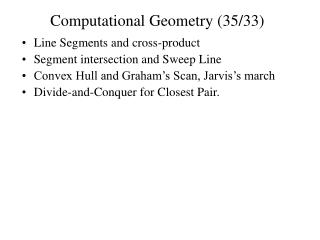DownloadDownload PresentationComputational Geometry (35/33)

# Computational Geometry (35/33)

Télécharger la présentation## Computational Geometry (35/33)

- - - - - - - - - - - - - - - - - - - - - - - - - - - E N D - - - - - - - - - - - - - - - - - - - - - - - - - - -
##### Presentation Transcript

1. Computational Geometry (35/33) • Line Segments and cross-product • Segment intersection and Sweep Line • Convex Hull and Graham’s Scan, Jarvis’s march • Divide-and-Conquer for Closest Pair.

2. Line Segments and cross-product (35.1/33.1) • A segment is a convex combination of ends • p1 = (x1,y1) and p2 = (x2,y2) • x =  x1+ (1-) x2, y =  y1+ (1-) y2 • p =  p1+ (1-) p2 • Cross product • p1  p2 = x1 y2 -x2 y1 • Vectors are collinear if p1 = c p2, cross-product=0 • The segment [p0p1] and [p0p2] turns left or right if (p1-p0)  (p2-p0) > 0 (<0) • Segment intersection: • bounding boxes • straddling

3. Segments and Sweep Line (35.2/33.2) • Sweep line is an imaginary vertical line moving from left to right • a1 is above a2 and a3 is above a2 a1 a3 a2

4. Convex Hull(35.3/33.3) • Convex Hull, CH(X), is the smallest convex polygon containing all points from X, |X|=n • Different methods: • incremental: moving from left to right updates CH(x1..xi). the runtime O(nlogn) • divide-and-conquer divides into two subsets left and right in O(n), then combine into one convex hull in O(n) • prune-and-search O(n logh), where h is the # points in CH uses pruning as for finding median to find upper chain

5. Graham’s Scan (35.3/33.3) O(nlogn)

6. Finding the Closest Pair(35.4/33.4) • Brute-force: O(n2) • Divide-and-conquer algorithm with recurrence T(n)=2T(n/2)+O(n) • Divide: divide into almost equal parts by a vertical line which divides given x-sorted array X into 2 sorted subarrays • Conquer: Recursively find the closest pair in each half of X. Let  = min{left, right} • Combine: The closest pair is either in distance  or a pair of points from different halves.

7. Combine in D-a-C (35.4/33.4) • Subarray Y’ (y-sorted) of Y with points in 2 strip • pY’ find all in Y’ which are closer than in  • no more than 8 in   2 rectangle • no more than 7 points can be closer than in  • If the closest in the strip closer then it is the answer 2 2   left right

8. Voronoi Graph • Voronoi region Vor(p) (p in set S) • the set of points on the plane that are closer to p than to any othe rpoint in S • Voronoi Graph VOR(S) • dual to voronoi region graph • two points are adjacent if their voronoi regions have common contiguous boundary (segment)

9. Voronoi Graph • Voronoi Graph in the rectilinear plane • Rectilinear distance: p = (x, y); p’=(x’,y’) Voronoi region of b ab b a bc c ac

10. THEOREM: For any set S of points in the plane, MST is subgraph of the Voronoi Graph VG(S) PROOF Let an edge XY between two points X and Y does not belong to the Voronoi graph VG(S). We will show that there is an X-Y- path in VG(S) which contains edges e1, e2,…,ek each shorter than XY, this will imply that XY not belong to MST. Indeed, for each edge eI (I=1,…,k) there is an MST path pI connecting ends of eI consisting of MST edges each no longer than eI. The path obtained by concatenating paths p1,…,pk connects X to Y and contains MST edges each shorter than XY. Thus XY does not belong to MST. Now we will find such X-Y-path e1, e2,…,ek in VG(S)

11. THEOREM: For any set S of points in the plane, MST is subgraph of the Voronoi Graph VG(S) PROOF Let an edge XY between two points X and Y does not belong to the Voronoi graph VG(S). We will show that there is an X-Y- path in VG(S) which contains edges e1, e2,…,ek each shorter than XY, this will imply that XY not belong to MST. Indeed, for each edge eI (I=1,…,k) there is an MST path pI connecting ends of eI consisting of MST edges each no longer than eI. The path obtained by concatenating paths p1,…,pk connects X to Y and contains MST edges each shorter than XY. Thus XY does not belong to MST. Now we will find such X-Y-path e1, e2,…,ek in VG(S)